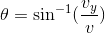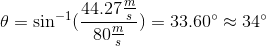# AP Physics C: Mechanics : Interpreting Work, Energy, and Power Diagrams

## Example Questions

### Example Question #11 : Work, Energy, And Power

An object with a mass of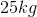is moving at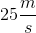in a straight line on a fricitonless surface. After a force of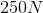acting in the direction of its motion is applied to it for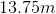, what is the object's speed in meters per second?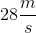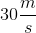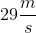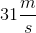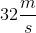Explanation:

Begin by using the following equation relating the initial and final kinetic energy and the work done on the object: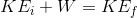Then, plug in the given variables and solve for the final speed.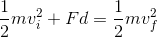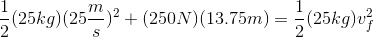Simplify terms.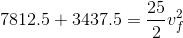Isolate the final velocity and solve.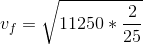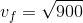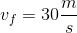### Example Question #133 : Mechanics Exam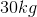projectile is launched straight upwards at an initial velocity of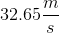. What is the maximum height that this projectile reaches in meters?

Round to the nearest meter, and assume the projectile encounters no air resistance.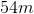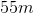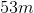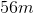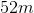Explanation:

You can use the motion equation and find the maximum, but it may be faster to use energy equations. Set the initial kinetic energy equal to the gravitational potential energy at the maximum height and solve for the height.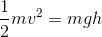Mass cancels.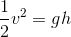Isolate the height and solve.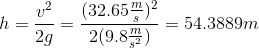Round to.

### Example Question #134 : Mechanics Exam

A set of cars on a roller coaster with a combined mass of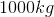is at the top of its initial hill and will drop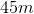down the hill before the track starts to rise again. What will the coaster's speed be at the moment the track starts to rise again?

Round to the nearest meter per second. You may also assume the track does not create friction.Explanation:

Remember that gravitational potential energy is not affected by the path downward (or upward)—whether it is straight, curved, or winding—only by how big the drop is. Once that is taken into account, you can simply set the initial gravitational potential energy and final kinetic energy equal to each other as if the coaster were falling straight down and solve for the final velocity.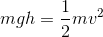The mass cancels.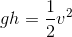Isolate the velocity and solve.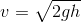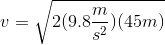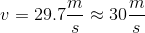### Example Question #11 : Work, Energy, And Power

A plane weighing 1500kg dives 40m with its engine off before it goes into a circular pattern with a radius of 200m while maintaining its speed at the end of its dive. How much centripetal force, in Newtons, is acting on the plane?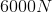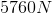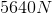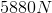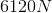Explanation:

First, find the gravitational potential energy of the drop. Then, set it equal to the kinetic energy at the end of the drop and solve for the velocity.The mass cancels.Isolate the velocity and solve.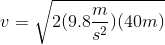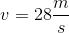This gives you the last term you need to solve for the centripetal force.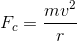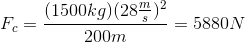### Example Question #136 : Mechanics Exam

If the maximum speed of an object attached to the end of a elastic has a 1:1 ratio (a meter per second for each meter) with how much the elastic is stretched or compressed from its starting position, which of the following is true?

None of these statements are true

The spring constant has a 4:1 ratio with the mass of the object in kilograms

The spring constant has a 2:1 ratio with the mass of the object in kilograms

The spring constant has a 1:2 ratio with the mass of the object in kilograms

The spring constant has a 1:1 ratio with the mass of the object in kilograms

The spring constant has a 1:1 ratio with the mass of the object in kilograms

Explanation:

Set the elastic potential and kinetic energy equations equal to each other: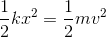You are given the fact that in this case,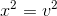. This allows you to simplify the equality.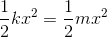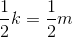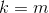This shows us that there is a 1:1 ratio between the spring constant of the elastic and the mass of the object.

### Example Question #137 : Mechanics Exam

A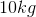pumpkin is being launched out of an air cannon. For safety reasons, the pumpkin cannot be more than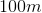off the ground during flight, and this particular cannon always launches pumpkins at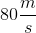meters per second—any more power and the pumpkin could be blasted apart; any less and the pumpkin may not leave the launch tube.

What is the maximum possible angle of launch in degrees?

Round to the nearest whole degree.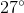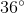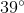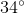Explanation:

We know the maximum height of the pumpkin, which tells us the maximum energy of the launch. Calculate the final gravitational potential energy.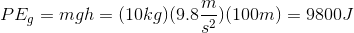Now set this value equal to a kinetic energy equation that uses the vertical component of the velocity at launch.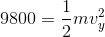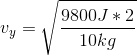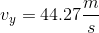Use the vertical velocity component to determine the launch angle.